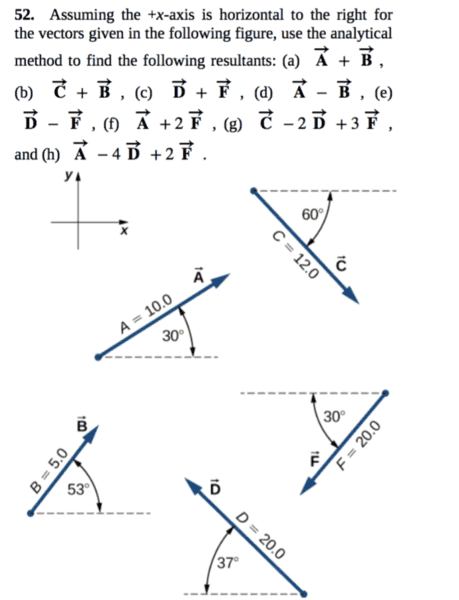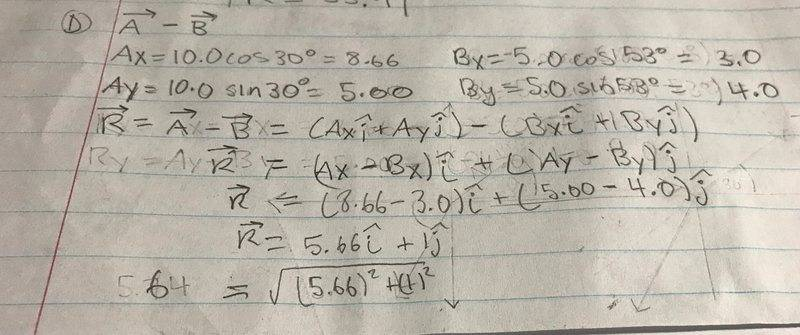# Algebra of Vectors: Find Difference & Multiply

• Sam Cepeda

## Homework Statement

[/B]## The Attempt at a Solution[/B]
I am not sure if this is the correct way of finding the difference between two vectors. I thought that if "B" was negative then it's direction would change as well as it's angle. If the angle changes then are my calculations wrong (I used original angle)? I was also curious to know if a similar process applies when multiplying two vectors?